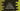# C++ program to compare two strings using memcmp## C++ program to compare two strings using memcmp:

In this post, we will learn how to compare two strings. We will use memcmp C library functions for that. It is defined in ctype header file. We will have to use c type strings, i.e. array of characters with this method.

I will show you the definition of memcmp and its uses with example.

## Definition of memcmp:

memcmp is defined as below:

``int memcmp ( const void * ptr1, const void * ptr2, size_t num );``

This method is actually used to compare two blocks of memory.

Here, ptr1 holds the address of the first block of memory and ptr2 holds the address of the second block of memory. It compares the first num bytes of blocks pointed by these pointers.

It returns one integer value. It can be 0, less than 0 or greater than 0.

• 0 means both are equal.
• greater than 0 means the first unequal byte is greater pointed by the first pointer than the second pointer.
• less than 0 means the first unequal byte is smaller pointed by the first pointer than the second pointer.

### Example of memcmp:

Let’s take a look at the below example program:

``````#include <iostream>
#include <cstring>
using namespace std;

int main()
{
char firstArr[] = "hello world";
char secondArr[] = "hello world";
int result = memcmp(firstArr, secondArr, 12);

cout << "result: " << result << endl;
return 0;
}``````

In this program, we are using memcmp to compare firstArr and secondArr and printing the result.

It will print:

``result: 0``

It prints 0 because both strings are equal. But, if we give different strings, the result will be different.

Let’s take a look at the below example:

``````#include <iostream>
#include <cstring>
using namespace std;

int main()
{
char firstArr[] = "hello World";
char secondArr[] = "hello woRld";
char thirdArr[] = "hello worlD";

int result = memcmp(firstArr, secondArr, 12);
int result2 = memcmp(thirdArr, secondArr, 12);

cout << "result: " << result << endl;
cout << "result2: " << result2 << endl;
return 0;
}``````

It will print:

``````result: -32
result2: 32``````
• For result, we are comparing firstArr with secondArr. The first different characters are W and w. ASCII value of W is 87 and w is 119. So, it returns 87 - 119 = -32.
• Similarly, for result2, we are comparing thirdArr with secondArr. The first different characters are r and R. ASCII value of r is 114 and R is 82. So, it returns 114 - 82 = 32.